Question

# A distribution of measurements is relatively mound-shaped with a mean of 80 and a standard deviation...

A distribution of measurements is relatively mound-shaped with a mean of 80 and a standard deviation of 14. Use this information to find the proportion of measurements in the given interval. Greater than 94

Given,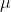= 80 ,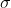= 14

We convert this to standard normal as

P(X < x) = P(Z < x -/)

So,

P(X > 94) = P(Z > 94 - 80 / 14)

= P(Z > 1 )

= 1 - P(Z < 1)

= 1 - 0.8413

= 0.1587

#### Earn Coins

Coins can be redeemed for fabulous gifts.

Similar Homework Help Questions
• ### 1. A distribution of measurements is relatively mound-shaped with a mean of 60 and a standard...

1. A distribution of measurements is relatively mound-shaped with a mean of 60 and a standard deviation of 11. Use this information to find the proportion of measurements in the given interval. between 49 and 71 2. A distribution of measurements is relatively mound-shaped with a mean of 80 and a standard deviation of 12. Use this information to find the proportion of measurements in the given interval. greater than 92 3. A distribution of measurements has a mean of...

• ### A distribution of measurements is relatively mound-shaped with mean 40 and standard deviation 10.

A distribution of measurements is relatively mound-shaped with mean 40 and standard deviation 10. (a) What approximate proportion of the measurements will fall between 30 and 50? (Enter your answer to two decimal places.) (b) What approximate proportion of the measurements will fall between 20 and 60? (Enter your answer to two decimal places.) (c) What approximate proportion of the measurements will fall between 20 and 50? (Enter your answer to three decimal places) (d) What approximate proportion of the measurements will be greater...

• ### Question 4 (10 points): A distribution of measurements is relatively mound-shaped with mean 45 and standard...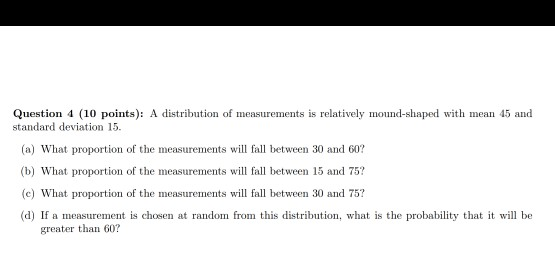Question 4 (10 points): A distribution of measurements is relatively mound-shaped with mean 45 and standard deviation 15 (a) What proportion of the measurements will fall between 30 and 60? (b) What proportion of the measurements will fall between 15 and 75? (c What proportion of the measurements w fall between 30 and 75? (d) If a measurement is chosen at random from this distribution, what is the probability that it will be greater than 60?

• ### 3. Consider the following. n = 5 measurements: 3, 3, 1, 2, 5 Calculate the sample variance, s2, using the definition formula.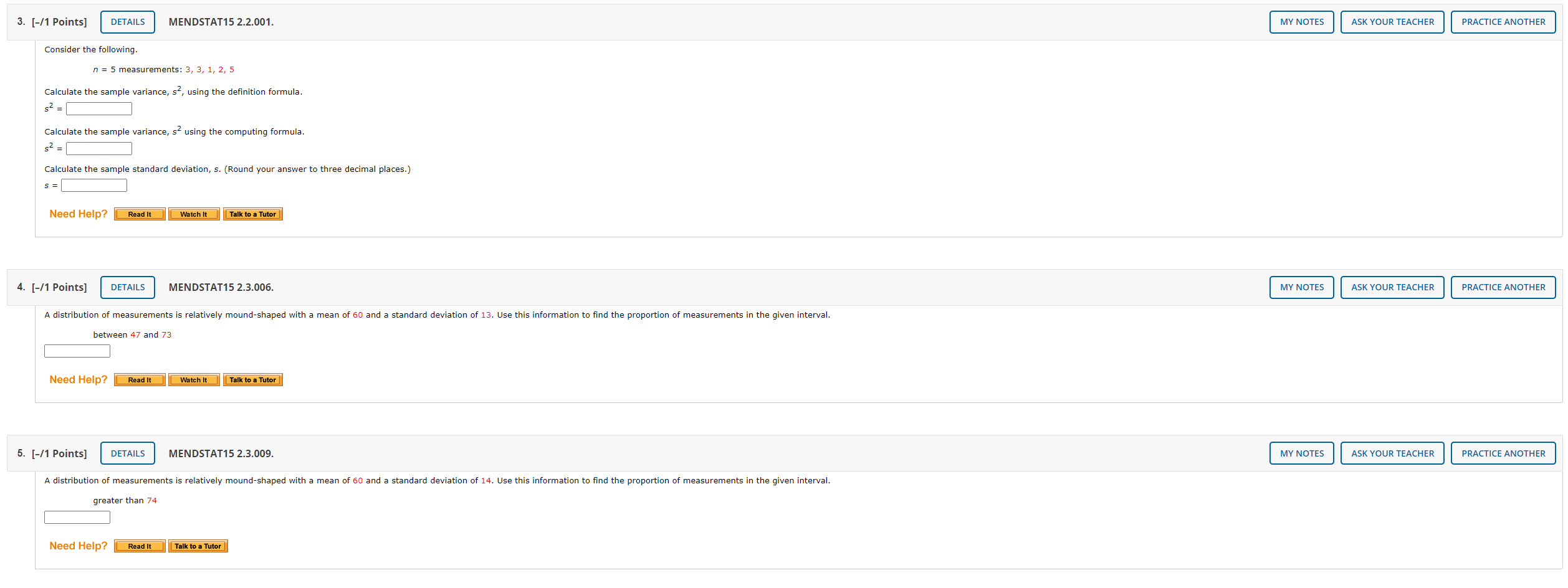3. Consider the following. n = 5 measurements: 3, 3, 1, 2, 5 Calculate the sample variance, s2, using the definition formula. Calculate the sample variance, s2 using the computing formula. Calculate the sample standard deviation, s. (Round your answer to three decimal places.)4. A distribution of measurements is relatively mound-shaped with a mean of 60 and a standard deviation of 13. Use this information to find the proportion of measurements in the given interval. between 47 and 73 5. A distribution of...

• ### please show your calculations A dlstrlbutlon of measurements Is relatlvely mound-shaped with mean 70 and standard...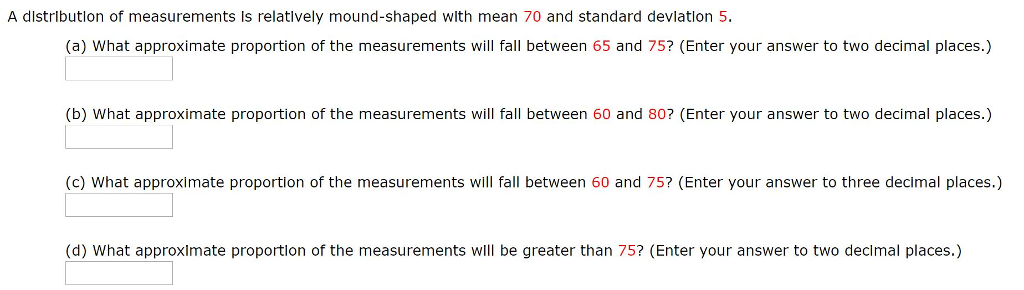please show your calculations A dlstrlbutlon of measurements Is relatlvely mound-shaped with mean 70 and standard devlation 5. (a) What approximate proportion of the measurements will fall between 65 and 75? (Enter your answer to two decimal places.) (b) What approximate proportion of the measurements will fall between 60 and 80? (Enter your answer to two decimal places.) (c) What approximate proportion of the measurements will fall between 60 and 75? (Enter your answer to three decimal places.) (d) What...

• ### The average first serve of a certain tennis player follows a symmetrical, mound-shaped distribution with a...

The average first serve of a certain tennis player follows a symmetrical, mound-shaped distribution with a mean of 122 and a standard deviation of 6.2 (miles per hour). Based on this information, approximately 95% of the first serves will fall between 115.8 and 128.2. True False

• ### 7. A sample dataset has a mound-shaped and symmetric distribution with mean of 57 and a...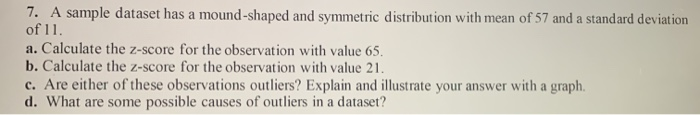7. A sample dataset has a mound-shaped and symmetric distribution with mean of 57 and a standard deviation of 11. a. Calculate the z-score for the observation with value 65. b. Calculate the z-score for the observation with value 21. c. Are either of these observations outliers? Explain and illustrate your answer with a graph, d. What are some possible causes of outliers in a dataset?

• ### Data are drawn from a bell-shaped distribution with a mean of 80 and a standard deviation...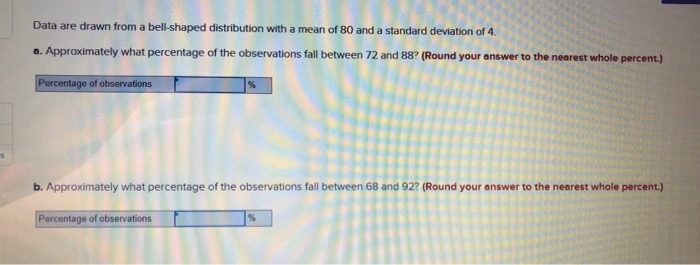Data are drawn from a bell-shaped distribution with a mean of 80 and a standard deviation of 4 a. Approximately what percentage of the observations fall between 72 and 88? (Round your answer to the nearest whole percent.) Percentage of observations b. Approximately what percentage of the observations fall between 68 and 92? (Round your answer to the nearest whole percent.) Percentage of observations c. Approximately what percentage of the observations are less than 76? (Round your answer to 1...

• ### Scores of an IQ test have a bell-shaped distribution with a mean of 100 and a standard deviation of 10

Scores of an IQ test have a bell-shaped distribution with a mean of 100 and a standard deviation of 10. Use the empirical rule to determine the following (a) What percentage of people has an IQ score between 70 and 1307 (b) What percentage of people has an IQ score less than 90 or greater than 110?  (c) What percentage of people has an IQ score greater than 120?

• ### The mean and standard deviation of test scores are 80 and 5 respectively. Assuming a bell-shaped...

The mean and standard deviation of test scores are 80 and 5 respectively. Assuming a bell-shaped distribution, what percentage of test-takers scored above 90?